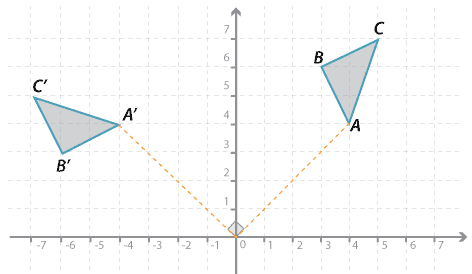### Rotating by 90 ° in an anticlockwise direction about the origin

In the diagram below, triangle $$ABC$$ is rotated 90 ° in an anticlockwise direction about the origin:

$$A(4, 4) \rightarrow A^\prime (–4, 4); B(3, 6) \rightarrow B^\prime (–6, 3); C(5, 7) \rightarrow$$

$$(–7, 5)$$.Detailed description